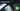# Hackerrank Solution of A Very Big Sum in Golang

August 26th, 2018
|

Solution for hackerrank warmup question a very big sum in golang. This question is very similar to simple array sum problem. The only difference being that the input array is huge. Let's tackle that in this blog post.

# Problem Statement

The question can be found at this link. The problem statement basically states that we need to print the sum of an array of integers whose length is provided as input. Couple of things to note here is

• We need to take array length as input
• In the next line, we are provided with an array of numbers, whose sum we need to print
• The integers in the array are huge ( `0<= i <= 10^10` )
• we are solving this in golang (assuming we know how to take input in golang, refer to my last post in case you need a refresher )

# Challenges

• Choose our preferred language as golang on hackerrank. The moment we do that, we get some 50-60 lines of code which are very unfamiliar to someone who is new to language.
• To solve this problem only thing we need to know is how to take input, show output, iterate over an array(kind of), and calculate sum.

# Solution

This is the template you get on hackerrank for this problem statement.

``````package main

import (
"bufio"
"fmt"
"io"
"os"
"strconv"
"strings"
)

// Complete the aVeryBigSum function below.
func aVeryBigSum(ar []int64) int64 {

}

func main() {

stdout, err := os.Create(os.Getenv("OUTPUT_PATH"))
checkError(err)

defer stdout.Close()

writer := bufio.NewWriterSize(stdout, 1024 * 1024)

checkError(err)

var ar []int64

for i := 0; i < int(arCount); i++ {
arItem, err := strconv.ParseInt(arTemp[i], 10, 64)
checkError(err)
ar = append(ar, arItem)
}

result := aVeryBigSum(ar)

fmt.Fprintf(writer, "%d\n", result)

writer.Flush()
}

if err == io.EOF {
return ""
}

return strings.TrimRight(string(str), "\r\n")
}

func checkError(err error) {
if err != nil {
panic(err)
}
}``````
• First go ahead and delete everything in the pre-existing template.
• Since the question is very similar to one of the previos ones, same code works for this one too. Refer to Simple Array Sum post for complete explation of the solution. So your solution should look something like this.
``````package main
import "fmt"

func main(){
var n,temp,sum int
fmt.Scan(&n)
for i:=0;i<n;i++{
fmt.Scan(&temp)
sum += temp
}
fmt.Println(sum)
}``````

The same code works here too, so what is all the fuss about long integers? Actually, although this code runs all well and good, the compiler is expecting a slightly different answer from you. An answer which would demonstrate that you understand that the concepts of memory and bits in a program. Now let me show you what I am talking about.

``````package main
import "fmt"

func main(){
var n,temp,sum int64
fmt.Scan(&n)
for i:=int64(0);i<n;i++{
fmt.Scan(&temp)
sum += temp
}
fmt.Println(sum)
}``````

Notice in this code snippet I made two changes

• In the variable declaration line, the variables are declared as int64 type
• `i:=0` is type casted to int64 type

Basically this explicitly tells the compiler to allocate 64bits of memory for these variables. Technically the range of n (length of array) is 1 to 10, so we could have specified int type for n and no type casting would have been required, or even a smaller signed type would have worked, with a type casting while initializing i.

Now the bigger question is why did the code with declaration of variables with `int` type work? The way golang works is int takes 32bit or 64bit depending on the platform. So if your platform running the code supported 64bit, int would have handled 64bit values and code would run just fine else it would fall back to 32bit. However if your platform doesn't support 64bit, int64 won't work either. So that is the reason both of the code snippets work and pass all the test cases.